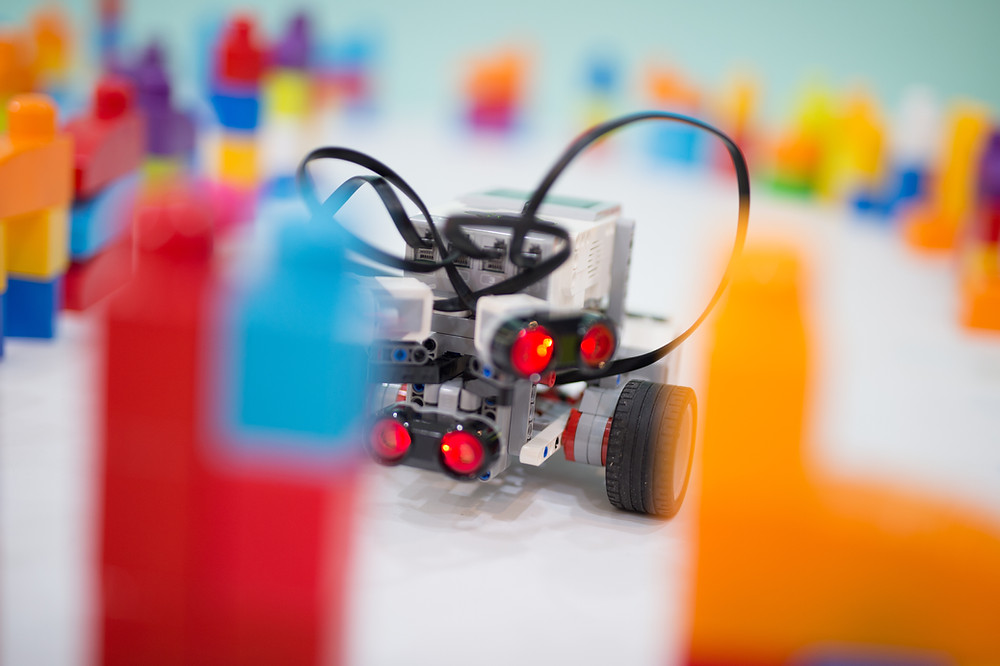# What exactly is coding logic?

We have written about why parents are now realizing the importance of coding and technology. (What is driving the increase in interest in coding for kids post-COVID?) With more parents searching for coding classes for their children, parents are asking us - what exactly is coding logic?

The concept of teaching coding logic is at the core of The Logic Coders. We strive to develop curriculum that is both useful and meaningful for students in the long-run.Coding Logic is solving problems with algorithms. This statement is deceptively simple.

For illustration, we will use the following problem: How do write a program that tells us if a given integer, n is a prime number? Try it out, think of a set of steps that when followed will always give us the answer to the problem.

One of the many algorithms to solve the problem:

1. Divide n by a divisor starting with 2

2. If there is no remainder from the division in step 2, stop and conclude that n is not a prime number

3. Else repeat from step 1, increasing the divisor by 1 each time until the last divisor is equal to n-1.

4. When the last divisor is equal to n-1, stop and conclude that n is a prime number

Now, challenge yourself to come up with an algorithm to find the largest prime number under 1,000. Even though we all know what prime numbers are, conceptualizing the algorithm requires mental gymnastics.

Being able to code is the ability the solve complex problems by providing a set of instructions to a computer

Coding is more than giving instructions to a computer. The most difficult part of coding is the ability to code is the ability to think critically about a problem breaking it down into steps and solve it.

But isn't learning to code learning Python, Java or another coding language? Take a look at the Computer Science Foundation modules at NUS. That is right. There are no specific coding languages there.

• CS1101S Programming Methodology

• CS1231S Discrete Structures

• CS2030S Programming Methodology II

• CS2040S Data Structures and Algorithms

• CS2100 Computer Organization

• CS2103T Software Engineering

• CS2106 Introduction to Operating Systems

• CS3230 Design and Analysis of Algorithms

The Logic Coders is a coding school for kids aged 6-16 in Singapore

Locations: City Square Mall #06-05 / Parkway Centre #13-07

We offer term-time and holiday programs using the Lego EV3 Robotics platform and Python